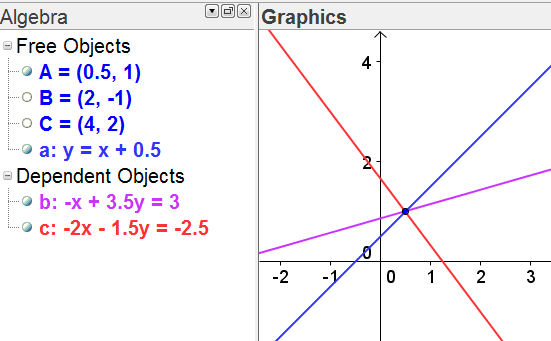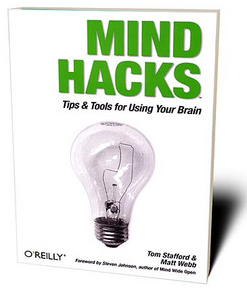# Notes to Self: Probability (Rules)People commonly use statistics like a drunk uses a lamppost:
for support, rather than for illumination.   - Mark Twain

Your tone of voice matters. Read the title of this post again... only this time, be really enthusiastic when your internal voice reads "Rules"

In my experience as a high school statistics teacher, probability is a topic that can prove vexing to even the 'best' students. The purpose of this post is for me to collect my thoughts on some things I am noticing in both my non-AP Statistics classes and in my AP Statistics classes. This may look like a random hodge-podge of my thoughts on teaching probability concepts... and it is. Empirically I have noticed a few things I think contribute to some of the misconceptions.

• When we introduce the Binomial setting, we talk about two possible outcomes. We often classify these outcomes as 'success' or 'failure.' However, if the context of the problem is mortality rates attributed to malaria, we wouldn't really consider death a 'successful' outcome. We would include a death as an outcome of interest if we were considering the proportion of deaths in the probability space attributed to malaria. This contributes to misunderstanding complementary events, too. I encourage students to think in terms of counting. What should we count? What should we not count? If we want to know the number of county residents that do NOT have red hair, what would be easier to count? Those without red hair? Or count those with red hair and take those away from the county population?• Student experience with Venn diagrams varies wildly. Some are skilled with drawing, labeling, and interpreting Venn diagrams; others not. My students often can draw a circle representing outcome A, and a circle representing outcome B, but they often forget to draw a boundary, like a rectangle, around the two circles to represent the probability space. Failing to draw some kind of boundary around the two circles causes students to forget to consider the case where neither A nor B occur. If the boundary is there, and either A or B are guaranteed to happen, then the student could simply write a "0" in the space outside A and outside B. [see above picture; no boundary given]. Students often struggle with labeling the probabilities corresponding to the interiors of each circle because they forget to address the outcome where both A and B occur simultaneously.
• Many students ask about "probability formulas." Think about how complicated Bayes Theorem first appears to students. Personally, when I work problems of this type, I make a tree diagram and label the outcomes. Somewhere along the way, students convince themselves there is always a formula for a probability problem. I think this reliance on formula is even more amplified than the same formula reliance we see in algebra and geometry classes.
• Students struggle with when to add probabilities and when to multiply probabilities. Some texts address these as the "General Addition Rule" or the "General Multiplication Rule." It's really interesting to watch students try to resolve cognitive dissonance - that is, when the student perceives their current understanding is disconfirmed by what they observe. One way I try to address this difference with students is to address disjoint outcomes and dependent outcomes. We go back to drawing Venn diagrams.
• Think about dice problems <ahem> I mean, random number cube problems. Suppose we are rolling two fair dice. We want to know the theoretical probability we roll a sum of five. My suspicion about a problem like this is the majority of students do not write out all 36 outcomes. Some might argue there is no need to write out all 36 outcomes because one can simplyconsider (1,4), (2,3), (3,2) and (4,1) to arrive at a neat and tidy 4/36... but I think this is a source of struggle for students that have a poor understanding of probability and counting. Many things might contribute to this avoidance of writing out all the possibilities. I imagine conserving class time and covering content might be reasons a teacher would avoid writing out all the cases... but the sample space provides the opportunity for rich discussion of many possible questions, not just the initial question.
• Students carry preconceived notions of equations and inequality statements. Try asking your high school students to write out a statement like
1/2 + x = (2/7)x + (6/7) = (-4/3)x + (5/3)
and ask students what they would do first to solve for x [the image of the graph of these three linear equations is below; I fabricated this example by picking a simple linear equation and crafting two additional lines where all three lines intersect at a common point]. Students have a similar lack of understanding of inequality statements. For example, suppose we have some random variable that is Normally distributed with mean 100 and standard deviation 15. Then the reason P(X < 85) and P(Z < -1) are equivalent is because we performed the same algebraic transformation to each quantity we originally compared. This transformation standardizes the original values and places them on a distribution with mean 0 and standard deviation 1. What I want my students to notice is when we perform the same algebraic transformations on the 'sides' of an inequality statement, the truth or falsehood of the statement remains unchanged.• Students also have trouble for similar reasons with statements where a random variable takes on a value between two endpoints. Suppose with the above distribution we consider P(70 < X < 85) and P(-2 < Z < -1) and why these two statements are equivalent.

A different perspective on the probability matter comes from one of my favorite books, Mind Hacks: Tips & Tools for Using Your Brain. The following is taken from pages 294 and 295. I encourage you to check out some of the practical knowledge in this book. Stafford and Webb offer terrific explanations for some of the rational and irrational things the human brain does.

Our ability to think about probabilities evolved to keep us safe from rare events that would be pretty serious if they did happen (like getting eaten) and to help us learn to make near-correct estimates about things that aren't quite so dire and at which we get multiple attempts (like estimating the chances of finding food in a particular part of the valley for example). So it's not surprising that, when it comes to formal reasoning about single-case probabilities, our evolved ability to estimate likelihood tends to fail us.

One example is that we overestimate low-frequency events that are easily noticed. Just ask someone if he gets more scared traveling in a car or by airplane. Flying is about the safe form of transport there is, whether you calculate it by miles flown or trips made. Driving is pretty risky in comparison, but most people would say that flying feels like the more dangerous of the two.

This site uses Akismet to reduce spam. Learn how your comment data is processed.